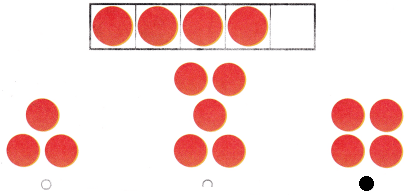# Texas Go Math Kindergarten Lesson 2.3 Answer Key Number in a Group

Refer to our Texas Go Math Kindergarten Answer Key Pdf to score good marks in the exams. Test yourself by practicing the problems from Texas Go Math Kindergarten Lesson 2.3 Answer Key Number in a Group.

## Texas Go Math Kindergarten Lesson 2.3 Answer Key Number in a Group

Explore

DIRECTIONS: Look at the soccer boils. Trace the number. Look at the baseballs. Write the number.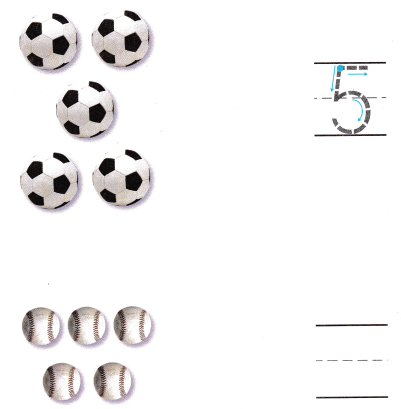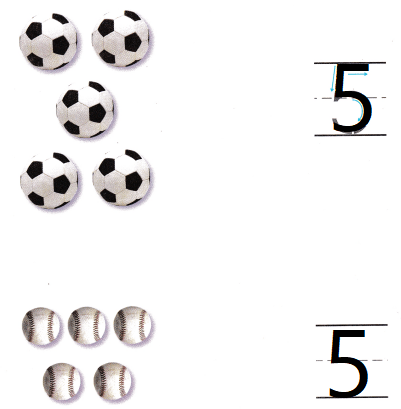Explanation:
There are 5 soccer’s in the first set and the 5baseballs in the second set.
Traced and written the number 5

Share and Show

DIRECTIONS: 1-2. Look at the sets. Write the number of objects you see in each set.

Question 1.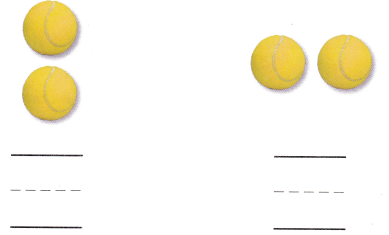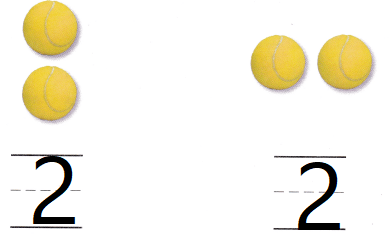Explanation:
There are 2 balls in the each set
Traced the number 2

Question 2.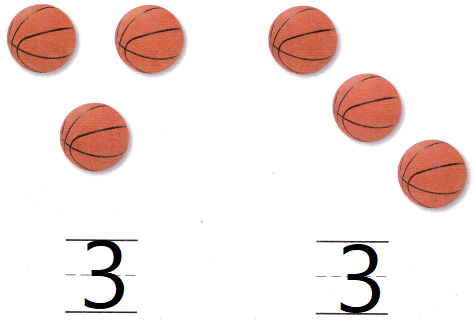Explanation:
There are 3 foot balls in each set
traced the number 3

DIRECTIONS: 3. Look at each set. Circle the sets that show 4. Write the number. 4. Look at each set. Circle the sets that show 5. Write the number.

Question 3.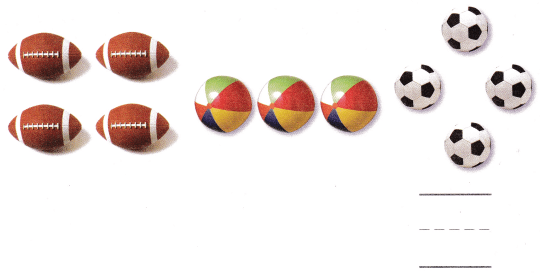Explanation:
In 2 of each set has 4 balls
circled then and written the number 4

Question 4.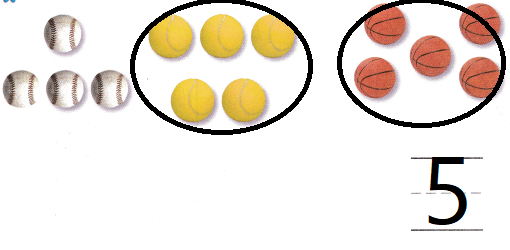Explanation:
In second, and third sets there are 5 in each set
circled and traced the number 5

DIRECTIONS: 5. Draw a set of 5 items. Then draw another set of 5 items in a different way. Write the number on the line. 6. Choose the correct answer. Which set shows the same number as the five frame?

Problem Solving

Question 5.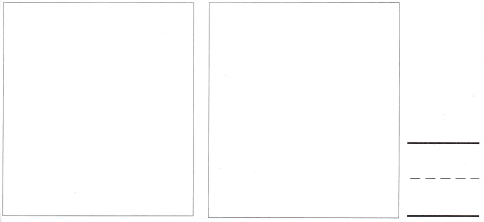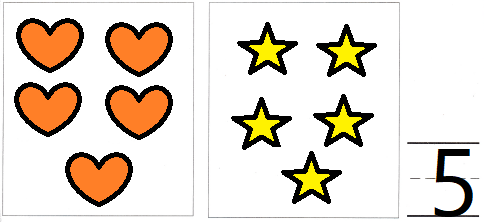Explanation:
Drawn a set of 5 hearts.
Then draw another set of 5 stars in a different way. Written the number on the line.

Question 6.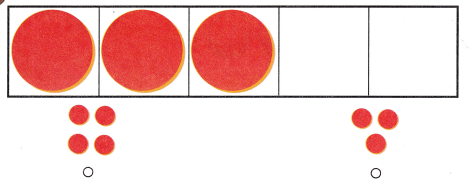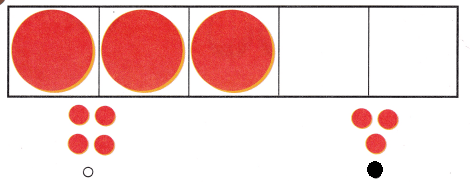Explanation:
There are three circles
and bubbled the option where the answer is 3

### Texas Go Math Kindergarten Lesson 2.3 Homework and Practice Answer Key

DIRECTIONS: 1-2. Look at the sets. Write the number of objects you see in each set.

Question 1.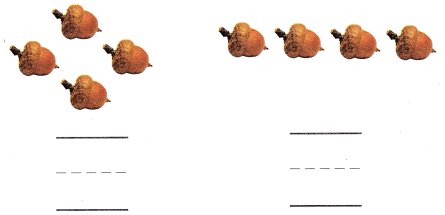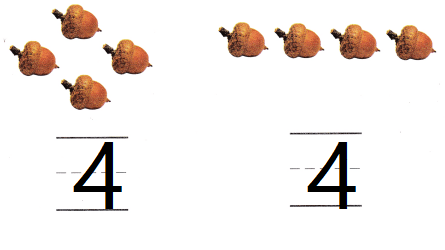Explanation:
there are 4 objects in each set
counted and written number 4

Question 2.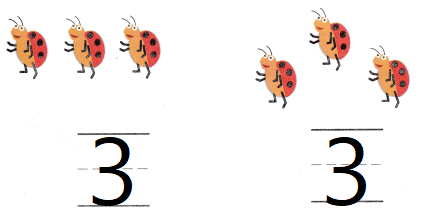Explanation:
there are 3 objects in each set
counted and written number 3

DIRECTIONS: Choose the correct answer. 3. Which set shows 3 daisies? 4. Which set shows 5 roses? 5. Which set shows the same number as the five frame?

Lesson Check

Question 3.Explanation:
Bubbled the set which has 3 daisies

Question 4.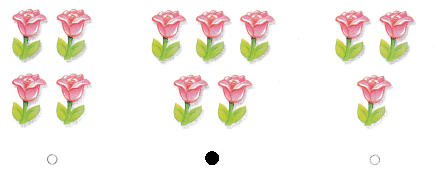Explanation:
Bubbled the set which has 5 roses

Question 5.